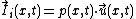# Sound Intensity

Also found in: Wikipedia.

## Sound intensity

A fundamental acoustic quantity which describes the rate of flow of acoustic energy through a unit of area perpendicular to the flow direction. The unit of sound intensity is watt per square meter. The intensity is calculated at a field point (x) as a product of acoustic pressure p and particle velocity u. Generally, both p and u are functions of time, and therefore an instantaneous intensity vector is defined by the equation below.The time-variable instantaneous intensity, , which has the same direction as , is a nonmoving static vector representing the instantaneous power flow through a point (x). See Power, Sound pressure

Many acoustic sources are stable at least over some time interval so that both the sound pressure and the particle velocity in the field of such a source can be represented in terms of their frequency spectra.

The applications of sound intensity were fully developed after a reliable technique for intensity measurement was perfected. Sound intensity measurement requires measuring both the sound pressure and the particle velocity. Very precise microphones for sound-pressure measurements are available.

An application of the intensity technique is the measurement of sound power radiated from sources. The knowledge of the radiated power makes it possible to classify, label, and compare the noise emissions from various pieces of equipment and products and to provide a reliable input into environmental design. See Sound

## Sound Intensity

the time-averaged energy transmitted by a sound wave through a unit area normal to the direction of wave propagation per unit time. In the case of periodic sound, averaging is done either over an interval of time, large in comparison with the period, or over an integral number of periods.

The sound intensity of a plane sinusoidal traveling wave is

I = pv/2 = p2/2pc

where p is the amplitude of sound pressure, v is the amplitude of sound velocity, p is the density of the medium, and c is the speed of sound in the medium. In a spherical traveling wave, the sound intensity is inversely proportional to the square of the distance from the source. In a standing wave, 1=0, that is, on the average there is no sound-energy flux.

Sound intensity is measured in the International System of Units in W/m2 and in the cgs system in erg/(sec • cm2) = 10-3 W/m2. Sound intensity is also evaluated by the intensity level according to the decibel scale; the number of decibels N = 10 log (I/I0), where I is the intensity of the given sound and I0 = 10-12 W/m2.

I. G. RUSAKOV

## sound intensity

[′sau̇nd in‚ten·səd·ē]
(acoustics)
For a specified direction and point in space, the average rate at which sound energy is transmitted through a unit area perpendicular to the specified direction.

## sound intensity

The average rate of sound energy transmitted in a specified direction through a unit area normal to this direction at the point considered.
References in periodicals archive ?
In future studies, it can be used three dimensional sound intensity measurement technique.
4 The incidence of this problem is directly dependent on sound intensity and exposure duration.
No studies to date have objectively assessed whether pinna morphology affects sound intensity detected within the external auditory canal (EAC).
Al Muhairi said: "The noise-monitoring radar consists of two integrated systems: the sound intensity sensor and an advanced camera that takes digital photos of the violating vehicles.
0]' (in sone/Bark) is the referenced specific loudness, E is the sound intensity in each critical band, [E.
Lehiste (1970) defined sound intensity as the square of the pressure variations of the sound wave.
A noise-measurement device on the outside wall of this box could measure the sound intensity that makes its way outside.
The amplitude of the sound pressure p is proportional to the sound intensity I.
OSHA did extensive sound intensity testing and came up with a time-weighted average of just over 86 decibels (dBA).
com)-- Since Japan has no large-scale acoustic underwater ranging system, JRC Tokki invested in a portable sound intensity system that enables field measurements to be made on large vessels.

Site: Follow: Share:
Open / Close